# Thermo-mechanical stress simulation of wire bonding using EMS

HOME / Applications / Thermo-mechanical stress simulation of wire bonding using EMS

### Applications

Used Tools:## Introduction

Wire bonding method is one of the most important interconnection techniques in electronics packaging. It is used to make electrical contact between integrated circuits or other semiconductor devices and the circuit board during device fabrication.
To evaluate the reliability of this interconnection technique in particular applications, an integrated electromagnetic, thermal and structural analysis is necessary.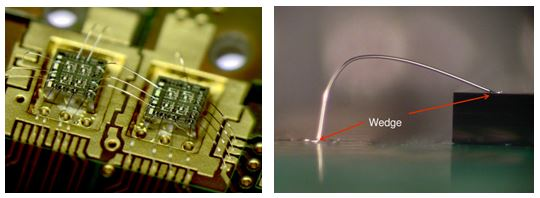Figure 1 - Wire bonding example 

## Problem description

An EMS Magnetostatic simulation coupled to thermo-structural analysis is performed to study the failure behavior of a wire bonding connection. The model studied consists of a 300 µm thick wire connecting two copper layers on a common aluminum substrate.

The wire loop geometry is illustrated in Figure 2a - the height H is the distance from the top of the wire to the substrate; the bond length L is defined as the foot to foot distance (dimension presented in Table 1). The present example aims to determine the magnitude of wire deflection in its critical high temperature zone.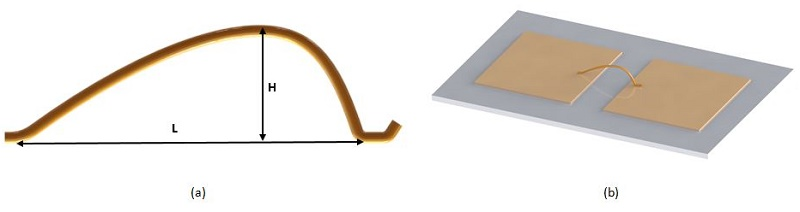Figure 2 - Wire bond (H: Height, L: length)  a).  3D Model b).

Table1 - Model dimensions 
 Dimensions Aluminum substrate Copper layer Wire Length(mm) 43 15 9 Height(mm) 1 0.3 3 Width(mm) 25 15 0.3 (Diameter)

## Simulation Setup

The Magnetostatic module of EMS, coupled to steady state thermal and structural analysis, is used to compute and visualize magnetic results, temperature distribution and mechanical deformation of the wire.

To perform an analysis using EMS, the following important steps need to be performed:

1. Select an appropriate material for all solid bodies.
2. Define the necessary electromagnetic inputs.
3. Apply the necessary thermal inputs.
4. Apply the structural boundary conditions.
5. Mesh the entire model and run the solver.

## Materials

In our case study, the following properties of material are used (Table 2):

Table 2 - Material properties

 Part Density (Kg/) Relative permeability Electrical conductivity (S/m) Specific heat capacity (J/Kg. K) Thermal conductivity (W/m. K) Elastic Modulus (Pa) Poisson’s ratio Thermal expansion coefficient (/K) Wire (Al-H11) 2700 1 3.57 E+07 897 230 71.8 E+09 0.33 25.3 E-06 Alumina substrate96%) 3690 1 0 687 24.7 Not required Copper layers 8900 0.99 5.7 E+07 390 385

## Electromagnetic Inputs

The wire is defined as a solid coil carrying a 10A DC current.

## Thermal Input

Initial temperature applied to both bonded feet of the wire is set to 300 K. The thermal convection inputs for the ambient air body:
-The initial (ambient) temperature of the simulation is set to 300 K
-Convection coefficient on the surrounding air is set to 10 W/m² K

## Mechanical boundary conditions

Fixed boundary conditions are applied to both feet of the bonded wire and to the four faces of each DCB substrate, as shown in the figure 3: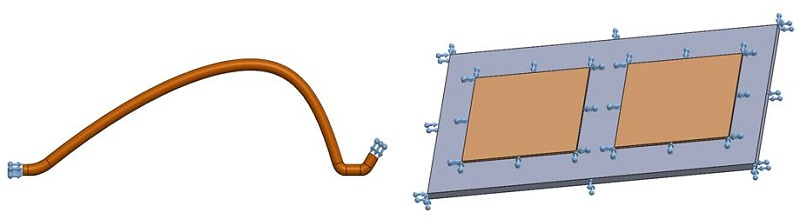Figure 3 - Applied mechanical boundary conditions

## Meshing

A mesh Control was applied on the wire for a finer meshing, as it is the most important component in the analysis, for a better results accuracy in this part, (Figure 4).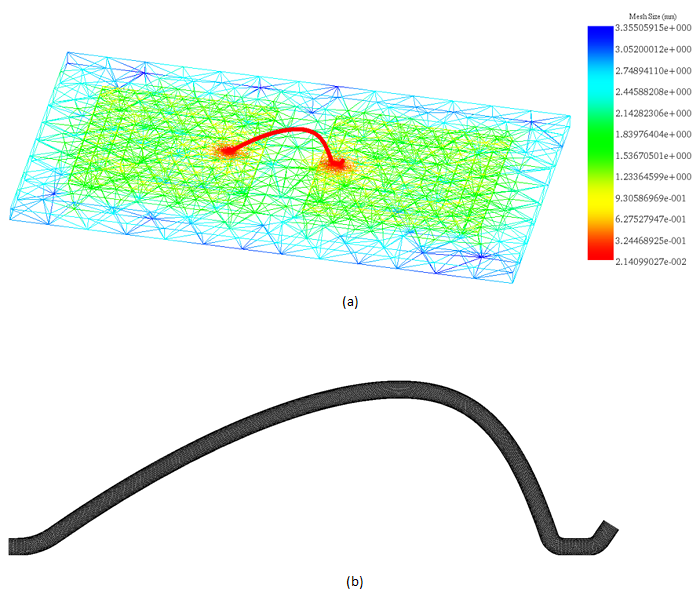Figure 4 - Mesh plot for the whole model a), the wire b).

## Results

The simulation results include: magnetic flux density, magnetic field intensity, applied current density, temperature and heat flux distribution, mechanical displacement, stress, etc.

EMS offers the possibility to visualize results as 3D plots and curves.  The figure below illustrates the distribution, in a vector plot, of the applied current density between the two bonded feet of the wire.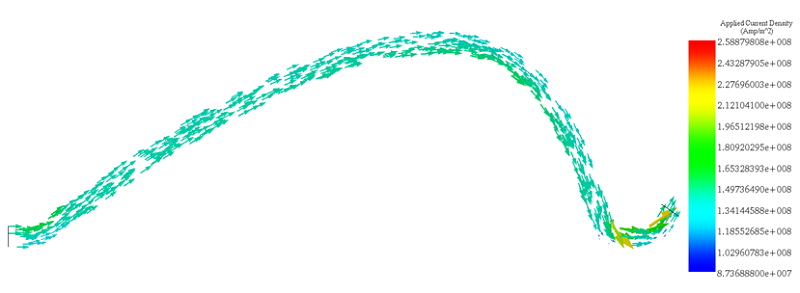Figure 5 - Vector plot of the applied current density.

The Table 3 contains the DC resistance of the simulated wire which is computed and generated by EMS in the results table.

Table 3 - Wire resistance

 Resistance (ohms) Solid Coil 4.8532 E-0.003

The Figure 6 shows the temperature distribution in the wire that achieves a maximum value of 340 K.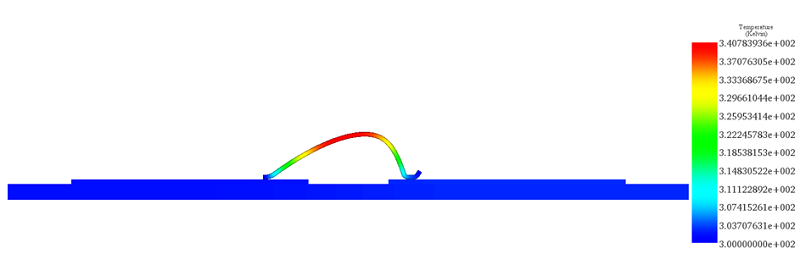Figure 6 - Fringe plot of temperature distribution

The maximum of temperature is located in the peak point of the wire due to the current concentration in this location. This high temperature zone will also experience the maximum mechanical deflection as shown in the Figure 7. Table 4 displays a comparison between EMS and reference results.

Table 4 - Comparative table between EMS and the reference  results

 EMS Reference  Temperature (Kelvin) 340 337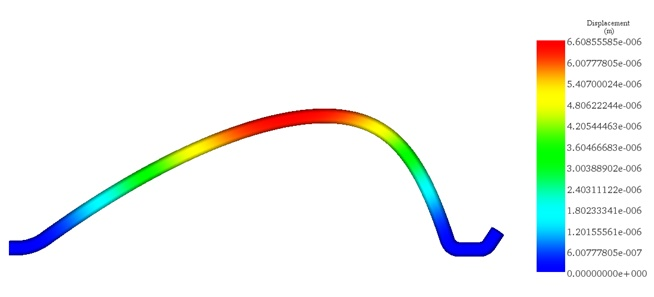Figure 7 - Resultant displacement plot

Figure 8 shows the displacement plot results generated by EMS, in the bonded wire, which correlate very well with the reference results.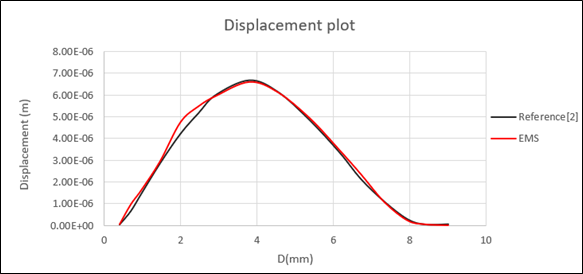Figure 8 - The wire displacement plot along the wire - EMS and Reference  results

## Conclusion

This example presents an EMS numerical evaluation of the performance of a bonding wire case. It enables a better estimation of the fatigue the wire will sustain during its lifetime. The obtained results show a very good agreement with the reference  for both structural and thermal analysis.

## References

. https://www.we-online.com/web/en/wuerth_elektronik/start.php
. Dagdelen, Turker. "Failure analysis of thick wire bonds. MS thesis". University of Waterloo, 2013.
. Dagdelen, Turker, Eihab Abdel-Rahman, and Mustafa Yavuz. "Reliability Criteria for Thick Bonding Wire." Materials 11.4 (2018): 618.